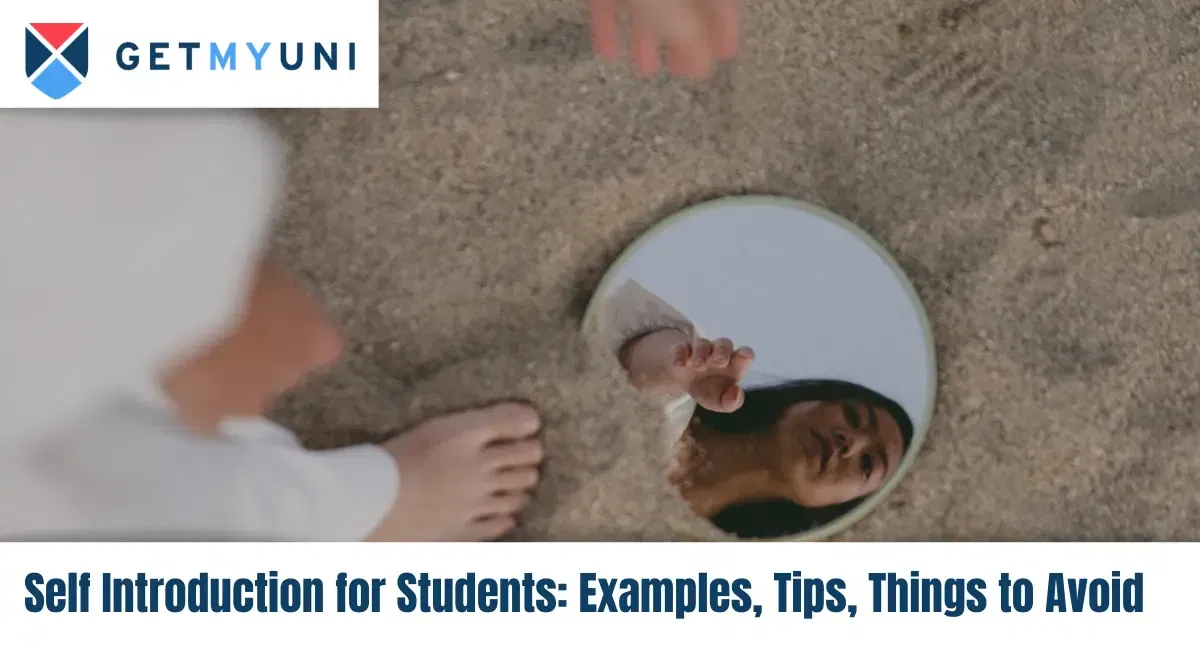# Self Introduction for Students: Examples, Tips, Things to AvoidOct 10, 2023

Share it on:

A self introduction for students is a concise, assured, and straightforward introduction. It should describe the student's personality, identity, and what details other people should be aware of.## FAQs

What is the time limit for self introduction for students?

How many points does a self introduction for students require?

What is the importance of Self Introduction for students?